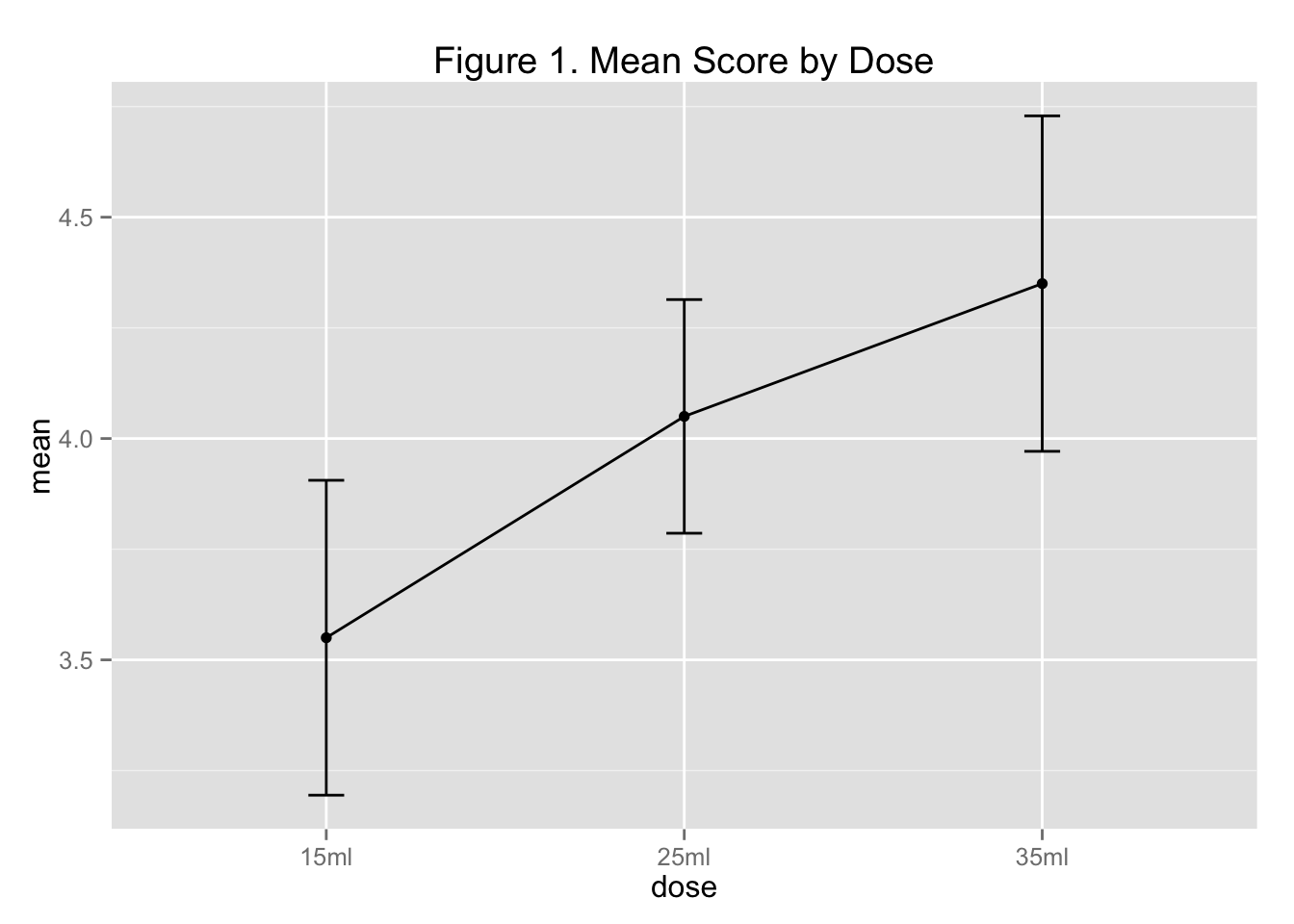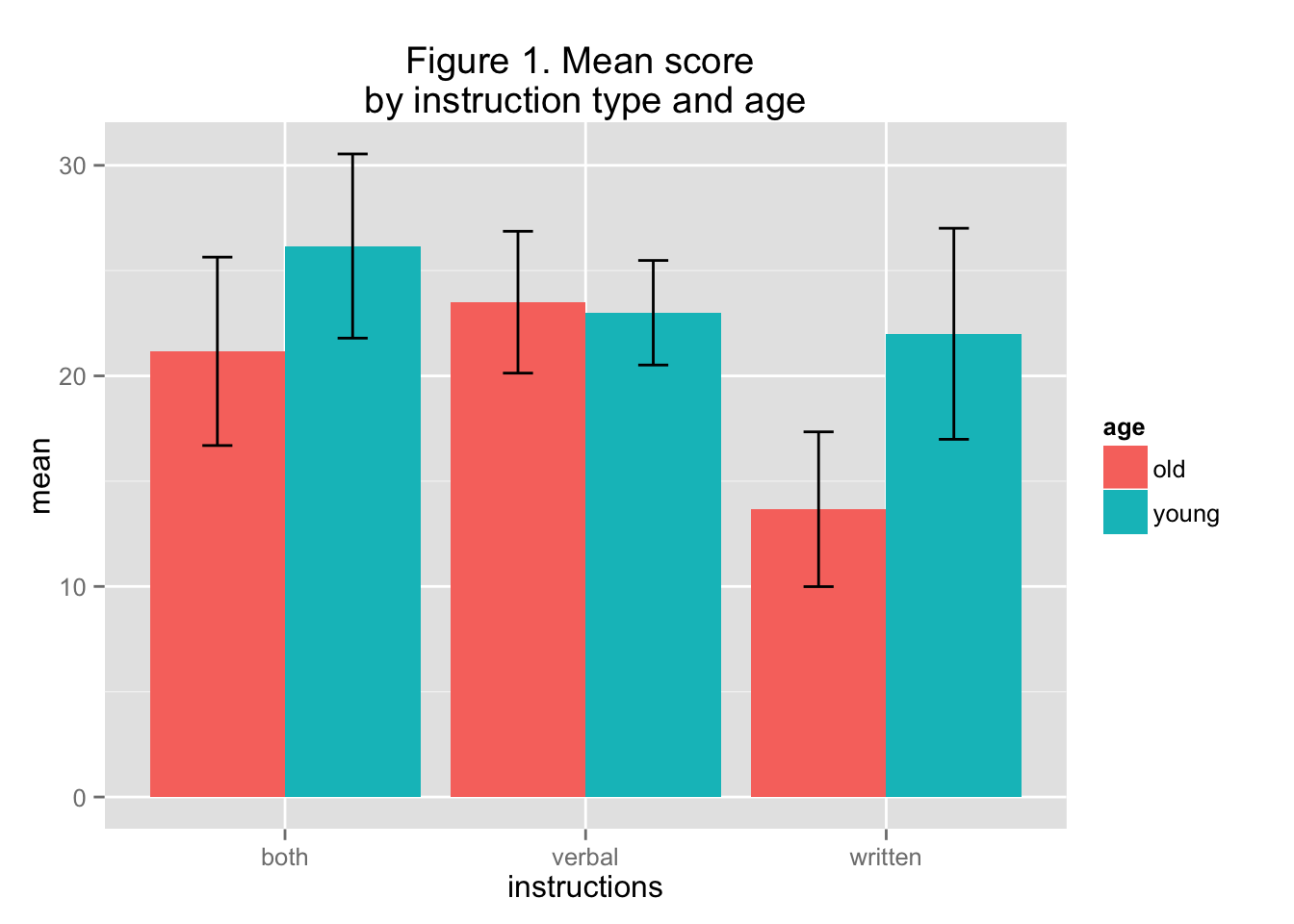# ANOVA + Contrasts in R

The linked Dropbox file has code and data files for doing contrasts and ANOVA in R.

https://www.dropbox.com/sh/132z6stjuaapn4c/AAB8TZoNIck5FH395vRpDYPPa?dl=0

R Club 11.3.15 ANOVA Contrasts

# set the working directory
setwd("/Users/jessicakosie/Dropbox/RClub_11.3.15")

# clear the environment
rm(list=ls())

## Planned Contrasts in R

# read in Data Set #1

# get descriptives
library(psych)
## Warning: package 'psych' was built under R version 3.2.2
describeBy(data$score, data$animal, mat=TRUE)
##    item   group1 vars  n mean        sd median trimmed    mad min max
## 11    1   Cougar    1 10  3.0 1.1547005    3.0   3.000 1.4826   1   5
## 12    2      Dog    1 10  9.1 0.9944289    9.0   9.250 1.4826   7  10
## 13    3 HouseCat    1 10  1.6 0.6992059    1.5   1.500 0.7413   1   3
## 14    4     Wolf    1 10  6.9 1.1972190    7.0   6.875 1.4826   5   9
##    range       skew   kurtosis        se
## 11     4  0.0000000 -0.9750000 0.3651484
## 12     3 -0.7809801 -0.5931107 0.3144660
## 13     2  0.5616761 -1.0787603 0.2211083
## 14     4  0.1678308 -1.1806760 0.3785939
# run an omnibus ANOVA

model <- aov(score ~ animal, data = data)
summary(model)
##             Df Sum Sq Mean Sq F value Pr(>F)
## animal       3  358.9  119.63   112.7 <2e-16 ***
## Residuals   36   38.2    1.06
## ---
## Signif. codes:  0 '***' 0.001 '**' 0.01 '*' 0.05 '.' 0.1 ' ' 1
# look at the levels of our factor

levels(data$animal) ##  "Cougar" "Dog" "HouseCat" "Wolf" # tell R which groups to compare c1 <- c(.5, -.5, .5, -.5) # canines vs. felines c2 <- c(1, 0, -1, 0) # cougars vs. housecats c3 <- c(0, 1, 0, -1) # dogs vs. wolves # combined the above 3 lines into a matrix mat <- cbind(c1,c2,c3) # tell R that the matrix gives the contrasts you want contrasts(data$animal) <- mat

# these lines give you your results
model1 <- aov(score ~ animal, data = data)

# can just look at the omnibus ANOVA (same as above)
summary(model1)
##             Df Sum Sq Mean Sq F value Pr(>F)
## animal       3  358.9  119.63   112.7 <2e-16 ***
## Residuals   36   38.2    1.06
## ---
## Signif. codes:  0 '***' 0.001 '**' 0.01 '*' 0.05 '.' 0.1 ' ' 1
# Make sure to use summary.aov here or 'split' might not work
summary.aov(model1, split=list(animal=list("Canines vs. Felines"=1, "Cougars vs House Cats" = 2, "Wolves vs Dogs"=3))) 
##                                 Df Sum Sq Mean Sq F value   Pr(>F)
## animal                           3  358.9   119.6 112.743  < 2e-16 ***
##   animal: Canines vs. Felines    1  324.9   324.9 306.188  < 2e-16 ***
##   animal: Cougars vs House Cats  1    9.8     9.8   9.236   0.0044 **
##   animal: Wolves vs Dogs         1   24.2    24.2  22.806 2.98e-05 ***
## Residuals                       36   38.2     1.1
## ---
## Signif. codes:  0 '***' 0.001 '**' 0.01 '*' 0.05 '.' 0.1 ' ' 1

## Polynomial Contrasts

### Plot the means

Before we run an ANOVA, let’s obtain a plot to get an idea of how the sample means differ.
Check out this useful tutorial: http://www.cookbook-r.com/Graphs/Plotting_means_and_error_bars_(ggplot2)/

# clear the environment

rm(list=ls())

# read in Data Set #2

# Lets take a look at the data - do we see a linear trend?

# Since we're not plotting the data themselves but rather means, first we need to calculate that, so we can feed it into the plot.
# To do that, we'll use a few functions from the dplyr package. Check out this tutorial: http://cran.rstudio.com/web/packages/dplyr/vignettes/introduction.html

library(dplyr) 
## Warning: package 'dplyr' was built under R version 3.2.2
##
## Attaching package: 'dplyr'
##
## The following objects are masked from 'package:stats':
##
##     filter, lag
##
## The following objects are masked from 'package:base':
##
##     intersect, setdiff, setequal, union
groups <- group_by(data, dose) # this just prepares it for us to calculate eveyrthing within each condition
plot.data <- summarise(groups,
mean = mean(score, na.rm=TRUE),
sd = sd(score, na.rm=TRUE),
n = n(),
se=sd/sqrt(n),
ci = qt(0.975, df=n-1)*se)
plot.data # take a peek
## Source: local data frame [3 x 6]
##
##     dose  mean        sd     n        se        ci
##   (fctr) (dbl)     (dbl) (int)     (dbl)     (dbl)
## 1   15ml  3.55 0.4972145    10 0.1572330 0.3556858
## 2   25ml  4.05 0.3689324    10 0.1166667 0.2639183
## 3   35ml  4.35 0.5296750    10 0.1674979 0.3789066
# ready to plot!
# line graph
library(ggplot2)
##
## Attaching package: 'ggplot2'
##
## The following object is masked from 'package:psych':
##
##     %+%
ggplot(plot.data, aes(x=dose, y=mean, group = factor(1))) +
geom_line() +
geom_point() +
geom_errorbar(aes(ymin=mean-ci, ymax=mean+ci), width=.1) +
ggtitle("Figure 1. Mean Score by Dose")### Polynomial Contrasts

For additional info on setting up contrasts (beyond the scope of this lab), check out the ever useful UCLA stats walkthrough

# run an ANOVA

results <- aov(score ~ dose, data=data)
summary.aov(results)
##             Df Sum Sq Mean Sq F value  Pr(>F)
## dose         2  3.267  1.6333   7.381 0.00277 **
## Residuals   27  5.975  0.2213
## ---
## Signif. codes:  0 '***' 0.001 '**' 0.01 '*' 0.05 '.' 0.1 ' ' 1
# Run a Polynomial Contrast

# Here's one way - tell R which groups to compare. These are the orthogonal polynomial contrasts.
c1 <- c(-1, 0, 1)
c2 <- c(0.5, -1, 0.5)

# combined the above 2 lines into a matrix
mat <- cbind(c1,c2)

# tell R that the matrix gives the contrasts you want
contrasts(data$dose) <- mat # these lines give you your results model1 <- aov(score ~ dose, data = data) summary.aov(model1, split=list(dose=list("Linear"=1, "Quadratic" = 2))) ## Df Sum Sq Mean Sq F value Pr(>F) ## dose 2 3.267 1.633 7.381 0.002773 ** ## dose: Linear 1 3.200 3.200 14.460 0.000744 *** ## dose: Quadratic 1 0.067 0.067 0.301 0.587607 ## Residuals 27 5.975 0.221 ## --- ## Signif. codes: 0 '***' 0.001 '**' 0.01 '*' 0.05 '.' 0.1 ' ' 1 # Try it using built-in contr.poly as well: # we tell R to get a polynomial contrast matrix for 3 conditions contrasts(data$dose) <- contr.poly(3)

# these lines give you your results
model2 <- aov(score ~ dose, data = data)
summary.aov(model2, split=list(dose=list("Linear"=1, "Quadratic" = 2)))
##                   Df Sum Sq Mean Sq F value   Pr(>F)
## dose               2  3.267   1.633   7.381 0.002773 **
##   dose: Linear     1  3.200   3.200  14.460 0.000744 ***
##   dose: Quadratic  1  0.067   0.067   0.301 0.587607
## Residuals         27  5.975   0.221
## ---
## Signif. codes:  0 '***' 0.001 '**' 0.01 '*' 0.05 '.' 0.1 ' ' 1
# Run a TukeyHSD Test

TukeyTest <- TukeyHSD(results)
TukeyTest
##   Tukey multiple comparisons of means
##     95% family-wise confidence level
##
## Fit: aov(formula = score ~ dose, data = data)
##
## \$dose
##           diff         lwr      upr     p adj
## 25ml-15ml  0.5 -0.02161703 1.021617 0.0621938
## 35ml-15ml  0.8  0.27838297 1.321617 0.0020820
## 35ml-25ml  0.3 -0.22161703 0.821617 0.3420861

## Factorial ANOVA

# clear the environment

rm(list=ls())

# read in Data Set #3

data <- read.csv("RClub_DataSet3_11.3.15.csv", header = TRUE)

### First, get a plot of your means, so you can see what’s going on.

# I'm choosing to plot with instruction type across the x-axis and grouped by age. It would also be fine to do it the other way around.

library(dplyr)
groups <- group_by(data, instructions, age) # this just prepares it for us to calculate everything within each combination of instructions  and age
plot.data <- summarise(groups,
mean = mean(score, na.rm=TRUE),
sd = sd(score, na.rm=TRUE),
n = n(),
se=sd/sqrt(n),
ci = qt(0.975,df=n-1)*se)

plot.data # take a peek
## Source: local data frame [6 x 7]
## Groups: instructions [?]
##
##   instructions    age     mean       sd     n        se       ci
##         (fctr) (fctr)    (dbl)    (dbl) (int)     (dbl)    (dbl)
## 1         both    old 21.16667 4.262237     6 1.7400511 4.472944
## 2         both  young 26.16667 4.167333     6 1.7013067 4.373348
## 3       verbal    old 23.50000 3.209361     6 1.3102163 3.368018
## 4       verbal  young 23.00000 2.366432     6 0.9660918 2.483418
## 5      written    old 13.66667 3.502380     6 1.4298407 3.675523
## 6      written  young 22.00000 4.774935     6 1.9493589 5.010986
# ready to plot!
# bar graph
# check this out: http://www.cookbook-r.com/Graphs/Plotting_means_and_error_bars_(ggplot2)/
library(ggplot2)
ggplot(plot.data, aes(x=instructions, y=mean, fill = age )) +
geom_bar(stat="identity", position=position_dodge()) +
geom_errorbar(aes(ymin=mean-ci, ymax=mean+ci), width=.2, position=position_dodge(.9)) +
ggtitle("Figure 1. Mean score \nby instruction type and age")### Run a 2 X 3 ANOVA on the data.

# The best way to run this is actually with the lm() command, not aov(). It stands for "linear model". ANOVAs, regressions, t-tests, etc. are all examples of the general linear model, so you can use this one command to do pretty much any of them in R.

# aov() works, and it will generate exactly the same source table for you (the math is all identical), but lm() gives you more useful output.

model <- lm(score ~ instructions*age , data=data)

# When you specify an interaction with *, R automatically assumes you want the main effects as well.
# So "instructions*age" is shorthand for "instructions + age + instructions*age".

anova(model) # to get a source table
## Analysis of Variance Table
##
## Response: score
##                  Df Sum Sq Mean Sq F value    Pr(>F)
## instructions      2 254.17 127.083  8.8150 0.0009741 ***
## age               1 164.69 164.694 11.4239 0.0020278 **
## instructions:age  2 119.39  59.694  4.1407 0.0258236 *
## Residuals        30 432.50  14.417
## ---
## Signif. codes:  0 '***' 0.001 '**' 0.01 '*' 0.05 '.' 0.1 ' ' 1
# We can discuss calculating simple effects in a later R Club